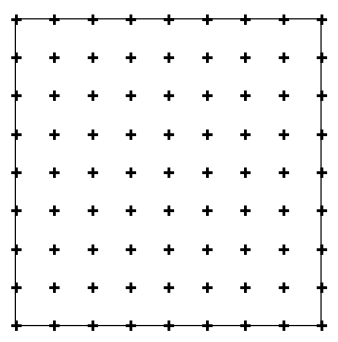시간 제한 메모리 제한 제출 정답 맞은 사람 정답 비율
2 초 512 MB 0 0 0 0.000%

## 문제

A rich man has a square room with mirrors for security or just for fun. Each side of the room is eight meters wide. The floor, the ceiling and the walls are not special; however, the room can be equipped with a lot of mirrors on the walls or as vertical partitions.

Every mirror is one meter wide, as tall as the wall, double-sided, perfectly reflective, and ultimately thin.

Poles to x mirrors are located at the corners of the room, on the walls and inside the room. Their locations are the 81 lattice points at intervals of one meter. A mirror can be fixed between two poles which are one meter distant from each other. If we use the sign "+" to represent a pole, the overview of the room can be illustrated as follows.Let us denote a location on the floor by (x, y) in a rectangular coordinate system. For example, the rectangular coordinates of the four corners of the room are (0,0), (8,0), (0,8) and (8,8), respectively. The location (x, y) is in the room if and only if the conditions 0 ≤ x ≤ 8 and 0 ≤ y ≤ 8 are satisfied. If i and j are integers satisfying the conditions 0 ≤ i ≤ 8 and 0 ≤ j ≤ 8, we can denote by (i, j) the locations of poles.

One day a thief intruded into this room possibly by breaking the ceiling. He stood at (0.75, 0.25) and looked almost toward the center of the room. Precisely speaking, he looked toward the point (1, 0.5) of the same height as his eyes. So what did he see in the center of his sight? He would have seen one of the walls or himself as follows.

• If there existed no mirror, he saw the wall at (8, 7.5).
• If there existed one mirror between two poles at (8, 7) and (8, 8), he saw the wall at (7.5, 8). (Let us denote the line between these two poles by (8, 7)-(8, 8).)
• If there were four mirrors on (8, 7)-(8, 8), (7, 8)-(8, 8), (0, 0)-(0, 1) and (0, 0)-(1, 0), he saw himself at (0.75, 0.25).
• If there were four mirrors on (2, 1)-(2, 2), (1, 2)-(2, 2), (0, 0)-(0, 1) and (0, 0)-(1, 0), he saw himself at (0.75, 0.25).

Your job is to write a program that reports the location at which the thief saw one of the walls or himself with the given mirror arrangements.

## 입력

The input contains multiple data sets, each representing how to equip the room with mirrors. A data set is given in the following format.

n
d1 i1 j1
d2 i2 j2
. . .
dn in jn

The first integer n is the number of mirrors, such that 0 ≤ n ≤ 144. The way the k-th (1 ≤ k ≤ n) mirror is fixed is given by dk and (ik, jk). dk is either 'x' or 'y', which gives the direction of the mirror. If dk is 'x', the mirror is fixed on (ik, jk)-(ik + 1, jk). If dk is 'y', the mirror is fixed on (ik, jk)-(ik, jk + 1). The end of the input is indicated by a negative integer.

## 출력

For each data set, your program should output the location (x, y) at which the thief saw one of the walls or himself. The location should be reported in a line containing two integers which are separated by a single space and respectively represent x and y in centimeter as the unit of length. No extra lines nor spaces are allowed.

## 예제 입력 1

0
1
y 8 7
4
y 8 7
x 7 8
y 0 0
x 0 0
4
y 2 1
x 1 2
y 0 0
x 0 0
-1


## 예제 출력 1

800 750
750 800
75 25
75 25